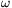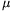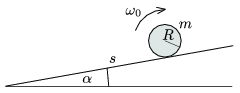Mathematical and Physical Journal
for High Schools
Issued by the MATFUND Foundation
 Already signed up? New to KöMaL?

#Problem P. 3991. (May 2007)

P. 3991. A solid cylinder of mass m and radius R is rotated with an angular speed of0 as shown in the figure and placed carefully to a long enough slope at a distance of s measured from the bottom of the slope. The incline angle is. How far from the bottom will the cylinder move and with what speed will it reach the bottom if

a=30o and=0.2;

b=20o and=0.4?Data: s=2 m, R=0.2 m,0=100 s-1.

(5 pont)

Deadline expired on June 11, 2007.

### Statistics:

 36 students sent a solution. 5 points: Almási 270 Gábor András, Balogh Máté, Blázsik Zoltán, Dudás János, Egyed Lenke, Egyed Zsombor, Hartstein Máté, Krämer Zsolt, Lovas Lia Izabella, Nagy 224 Csaba, Szilágyi Zsombor, Szolnoki Lénárd, Tűri Balázs, Tüzes Dániel, Varga Bonbien. 4 points: Almasi Susann Melinda, Csányi Gergely, Pálovics Róbert. 3 points: 5 students. 2 points: 3 students. 1 point: 2 students. 0 point: 6 students. Unfair, not evaluated: 2 solutionss.

Problems in Physics of KöMaL, May 2007